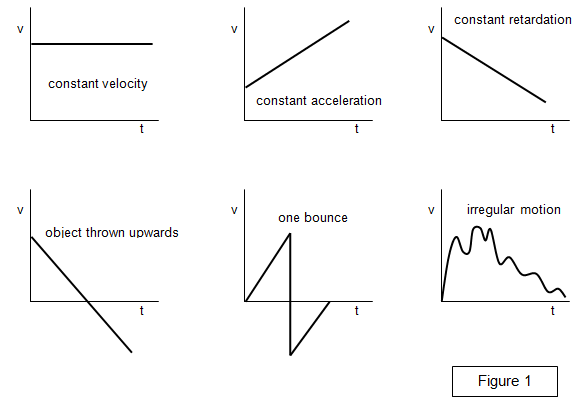# Velocity-time graphs

The set of graphs in Figure 1 show how the velocity varies with time for several different situations.The area below the line in each graph still represents the distance travelled in a certain time, whether the acceleration is uniform or not.
The slope of the line at any point (dv/dt) gives the instantaneous acceleration.

The average acceleration is found by dividing the velocity change by the time taken.

A VERSION IN WORD IS AVAILABLE ON THE SCHOOLPHYSICS USB

© Keith Gibbs 2020